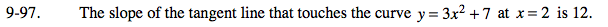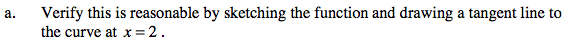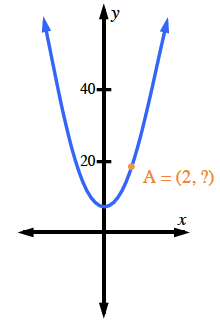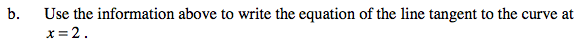### Home > PC > Chapter 9 > Lesson 9.3.1 > Problem9-97

9-97.
1. The slope of the tangent line that touches the curve y = 3x2 + 7 at x = 2 is 12. Homework Help ✎

1. Verify this is reasonable by sketching the function and drawing a tangent line to the curve at x = 2.

2. Use the information above to write the equation of the line tangent to the curve at x = 2.Use point-slope form.